# Discussion by Recognition Network

Network of members that are discussed with by recall

## Examples

### Basic Examples

Retrieve the graph:

 In:=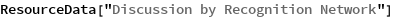Out=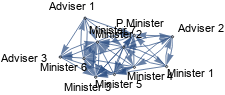Summary properties:

 In:=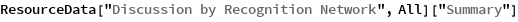Out=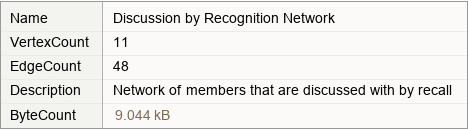### Basic Applications

Show the discussions between members:

 In:=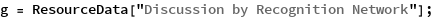In:=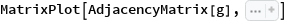Out=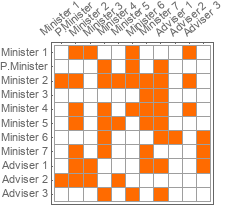Wolfram Research, "Discussion by Recognition Network" from the Wolfram Data Repository (2019)